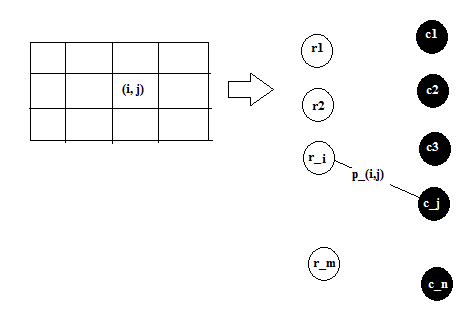Maximum matchings in Bipartite graphs is a very common problem in Computer Science and has an efficient solution using augmenting paths. The basic idea is to find alternating chains where we can increasing the matching iteratively. There are several problems that can be thought in terms of bipartite graphs. I’ll walk you through the detailed solution on one such problem in this post.

Let us assume that we have \$\$m times n\$\$ a grid with and a set S of points, each point placed at some grid intersection and we want to select the largest subset \$\$S_0 subseteq S\$\$ such that no two points of \$\$S_0\$\$ share the same row or the same column on the grid.

In order to derive a solution to the problem, let us first try to analyse the problem in order to break it into something we are already familiar with. We have been given an \$\$m * n\$\$ grid and some points at certain positions in the grid, \$\$(i,j)\$\$, \$\$1 leq i leq m\$\$ and \$\$1 leq j leq n\$\$. If we have already picked a point at an index \$\$(i_1,j_1)\$\$, then we can not pick another point \$\$(i_1,j_2)\$\$ or \$\$(i_2,j_1)\$\$. This means that we are trying to pick points that have none of the indices in common. We can model this problem as a bipartite matching problem by modelling the \$\$m\$\$ rows as one set of vertices of the graph and \$\$n\$\$ columns as another set of vertices of the graph.

Therefore, we construct a bipartite graph, \$\$G = (L,R,E)\$\$ where \$\$L = {u_1,u_2,...,u_m}\$\$ represents the rows of the grid and \$\$R = {v_1,v_2,...,v_n}\$\$ represents the columns of the grid. Now, we can model the edges in the bipartite graph, \$\$E\$\$ as a connection between the rows and columns in the grid. This connection in our grid is the presence of a point in the grid. Therefore, for every point , \$\$p in S\$\$ at position \$\$(i,j)\$\$ in the grid, we can make an edge from \$\$u_i\$\$ to \$\$v_j\$\$ in the bipartite graph. Note that the graph is indeed a bipartite graph because there can be no edge between two rows or two columns.Now, this problem is reduced to finding the maximum matching in a bipartite graph. A maximal matching will give a set of edges, \$\$E' subseteq E\$\$. Since, we have already mentioned that all the points in the set \$\$S\$\$ are modeled as edges in the bipartite graph, the set of edges, \$\$E'\$\$ actually represents a set \$\$S' subseteq S\$\$ of points in the grid. Another property of maximum matching is that the edges that it selects are vertex disjoint. Now, we can make an analogy with our grid. Since the vertices in the bipartite graph represent rows and columns in the grid, and since all the edges (\$\$in E'\$\$) are vertex disjoint (i.e. don't have any vertex in common), we can say that none of the vertices \$\$in S'\$\$ have a row or a column in common. Therefore, we have selected a set of vertices from the grid such that no two of them are in the same row or column. Now, since we have obtained this set \$\$S'\$\$ by applying a maximum matching algorithm on the bipartite graph, the set \$\$S'\$\$ that we obtain should be the biggest such subset possible.

However, let us also present a formal proof the above arguments. A formal proof will contain the following points.

• Every subset \$\$S' subseteq S\$\$ with points having unique rows and columns can be represented as a matching in the graph \$\$G\$\$. In order to prove this, let us consider a point in \$\$S'\$\$. This point \$\$p = (l,r)\$\$ can modelled as an edge from \$\$L\$\$ to \$\$R\$\$ in our \$\$G\$\$. Since, by the property of \$\$S'\$\$, there is no other point \$\$p' = (l',r')\$\$ with the same row and column, i.e. \$\$l' neq l\$\$ and \$\$r' neq r\$\$. Therefore, every point \$\$p in S'\$\$ can be modelled as an edge from \$\$L\$\$ to \$\$R\$\$ and such edges will have no vertex in common. This represents a matching in graph \$\$G\$\$.

• A matching (\$\$M\$\$) in graph \$\$G\$\$ represents a valid subset \$\$S' subseteq S\$\$ with points in unique rows and columns. This is exactly the inverse of the above and can be proved in a similar fashion. Consider an edge \$\$e = (l,r) in M\$\$. Since, \$\$M\$\$ is a matching, we can say that there will be no edge \$\$e' = (u',v') in M\$\$ such that \$\$u' = u\$\$ or \$\$v' = v\$\$. Now, if we model the \$\$e\$\$ as points, then the set of such points will not have any row or column in common. Hence we have proved that every matching in \$\$G\$\$ represents a valid subset \$\$S'\$\$.

• Finally, we need to prove that the maximum matching in \$\$G\$\$ corresponds to a maximum set \$\$S'\$\$ of row and column disjoint points. We will prove this by contradiction. Consider that we have obtained a maximum matching \$\$M\$\$ from \$\$G\$\$ such that there exists a set \$\$S'\$\$ which has more points than represented in the \$\$M\$\$, i.e. \$\$|S'| > |M|\$\$. Now, from first proof above, we can model \$\$S'\$\$ as a matching in \$\$G\$\$. Now, the size of this matching will be \$\$|S'|\$\$ and since this wasn't obtained by our maximum matching algorithm , therefore, \$\$|S'| leq |M|\$\$. This is a contradiction to our original assumption. Therefore, the maximum matching found by our algorithm produces the correct set of points \$\$S'\$\$.